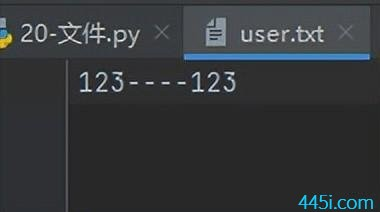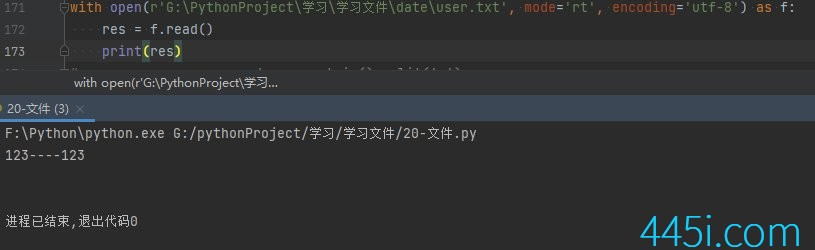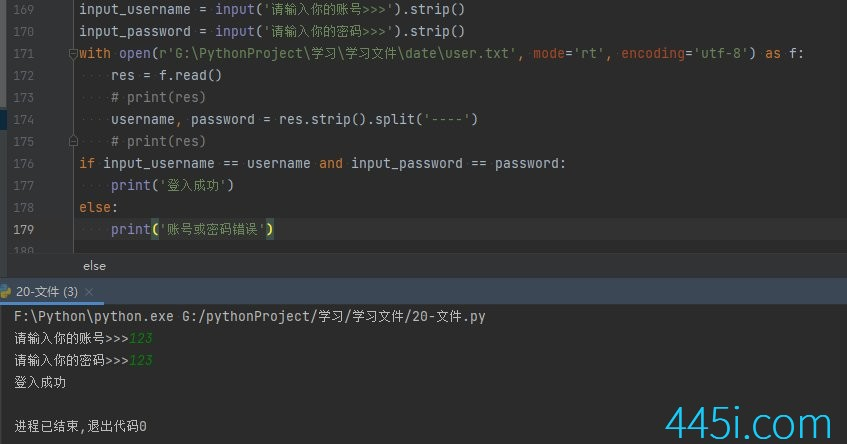# Python 登入案例优化

``````username = '123'

``````username = '123'

``````if input_username == username and input_password == password:
print('登入成功')
else:
print('账号或密码错误')``````

``````username = '123'
print('登入成功')
else:
print('账号或密码错误')````````````input_username = input('请输入你的账号>>>').strip()
with open(r'G:\PythonProject\学习\学习文件\date\user.txt', mode='rt', encoding='utf-8') as f:
print('登入成功')
else:
print('账号或密码错误')````````````with open(r'G:\PythonProject\学习\学习文件\date\user.txt', mode='rt', encoding='utf-8') as f:
print(res)
print(res)``````

``````input_username = input('请输入你的账号>>>').strip()
with open(r'G:\PythonProject\学习\学习文件\date\user.txt', mode='rt', encoding='utf-8') as f:
print('登入成功')
else:
print('账号或密码错误')``````暂无内容！

### 觉得文章有用就打赏一下文章作者

#### 支付宝扫一扫打赏#### 微信扫一扫打赏